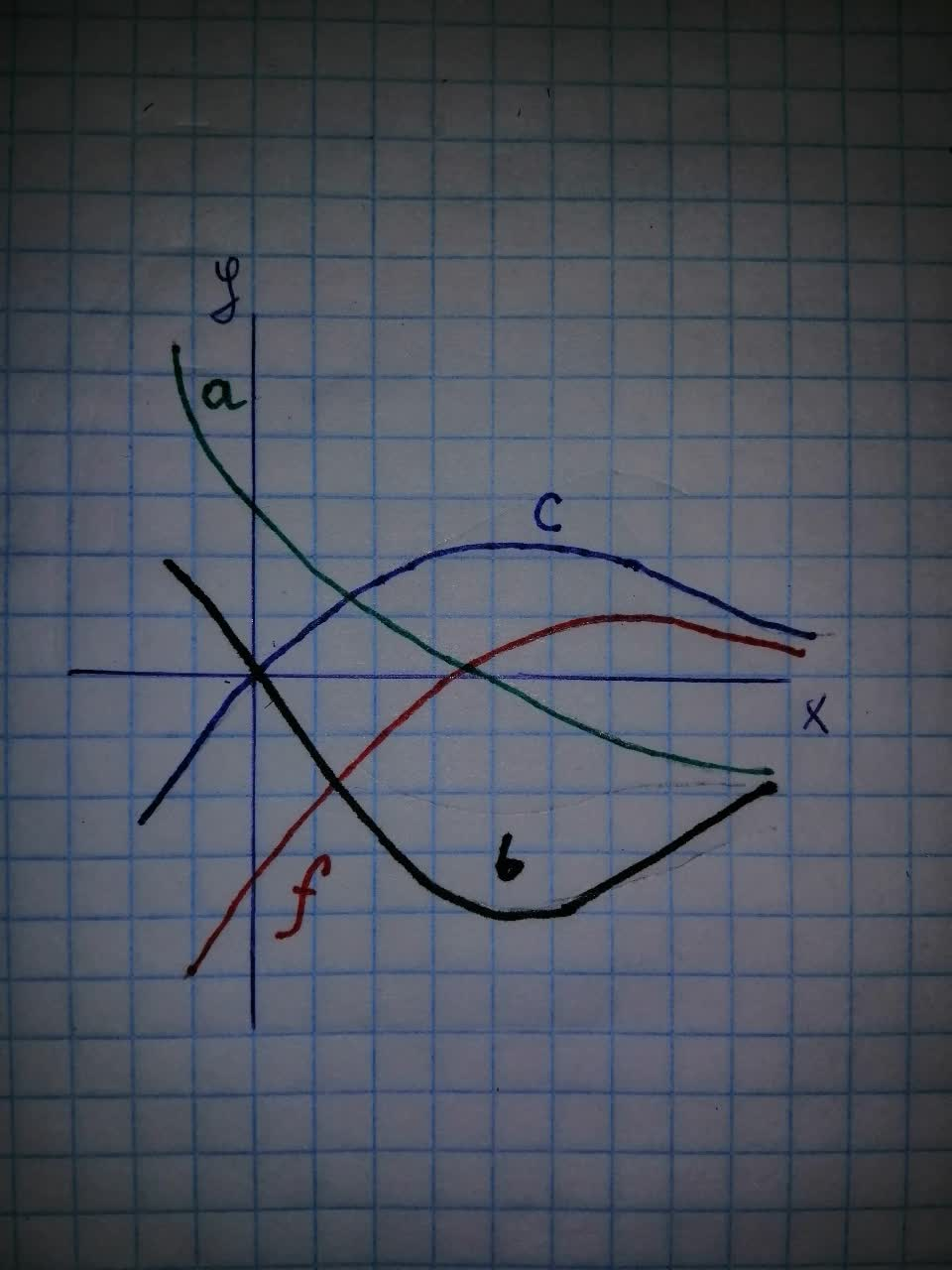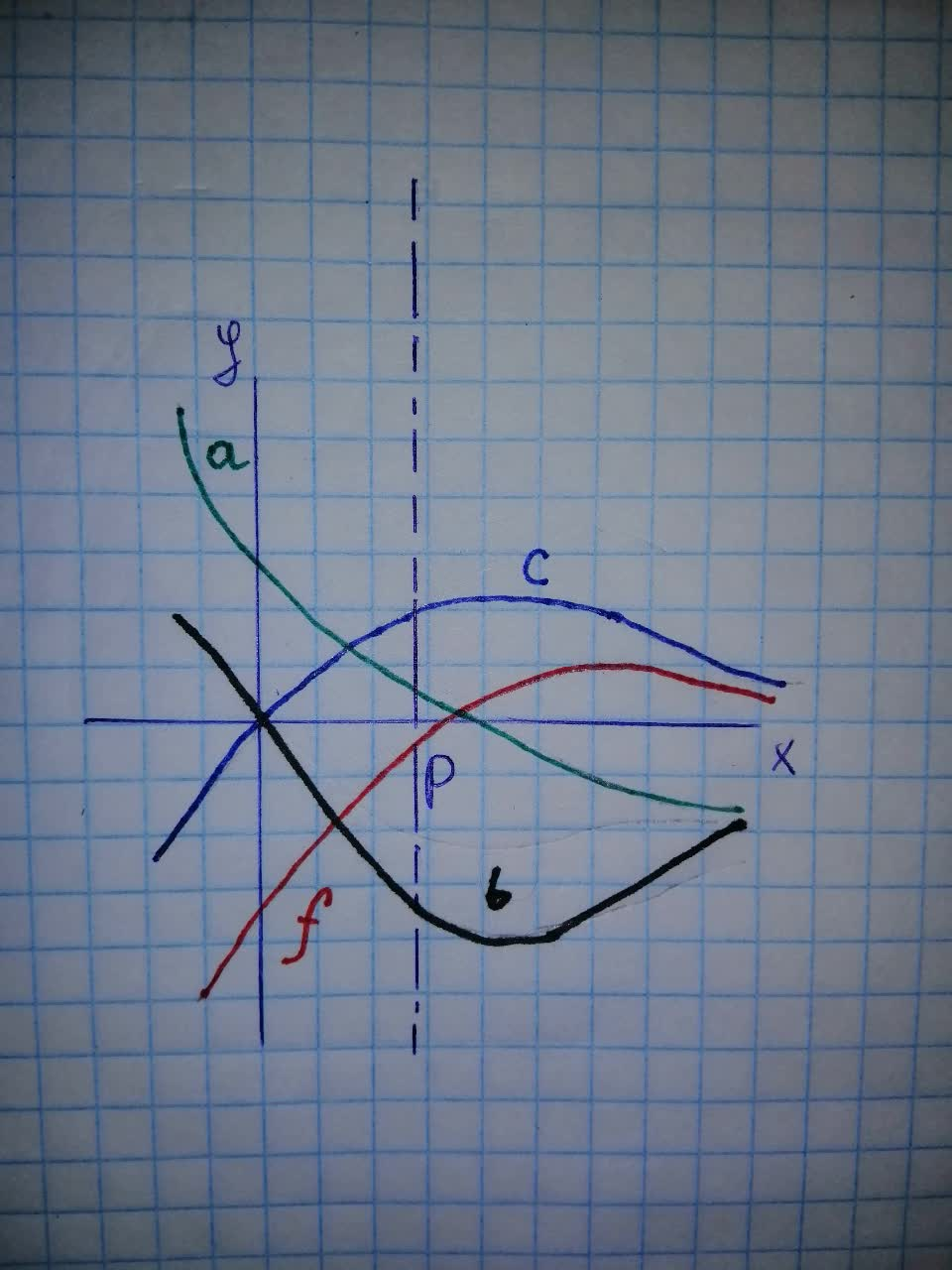# The graph of a function f is shown. Which graph is an antiderivative of f? Fphilosphy111of 2021-11-18 Answered
The graph of a function f is shown. Which graph is an antiderivative of f?You can still ask an expert for help

• Questions are typically answered in as fast as 30 minutes

Solve your problem for the price of one coffee

• Math expert for every subject
• Pay only if we can solve itColdst
Step 1
Let $g\left(x\right)$ be the antiderivative of $f\left(x\right)$. Then we must have
$g\left(x\right)=\int f\left(x\right)dx$
$⇒{g}^{\prime }\left(x\right)=f\left(x\right)$
Now, since $f\left(x\right)$ is negative that means the ${g}^{\prime }\left(x\right)$ is negative in this interval. So, g(x) (the antiderivative of $f\left(x\right)$ must be decreasing in this interval. This scenario is shown in two graphs a and b.
Again we notice that $f\left(x\right)$ is positive that means, ${g}^{\prime }\left(x\right)$ is positive in . So, the function $g\left(x\right)=$ antiderivative of $f\left(x\right)$ must be increasing in this interval and from the graph we can see that the graph b is increasing after the point $x=p$.
So, the correct graph for the antiderivative of $f\left(x\right)$ is b.